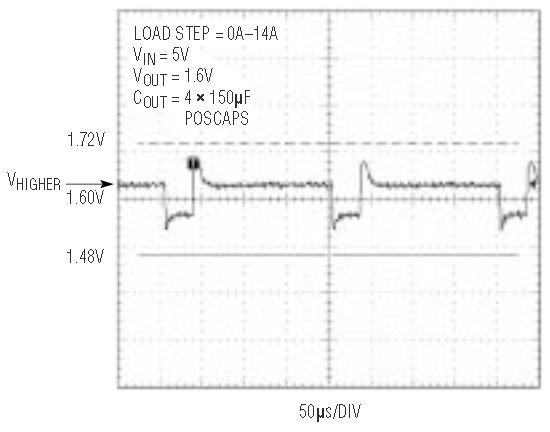Active Voltage Positioning Saves Output Capacitors in Portable Computer Applications

Introduction

Active voltage positioning is a technique that can be used to save cost and space by reducing the number of output capacitors required to meet a microprocessor’s power supply requirements. Total system cost and required PCB space are important aspects of today’s portable equipment designs, so decreasing the number of large, expensive output capacitors is worth some effort. Both the LTC1735/LTC1736 current mode switching regulator controllers (Linear Technology IX:1, February 1999, pp. 1, 3–5, 35) and the LTC1702/LTC1703 voltage mode controllers (Linear Technology IX:3, September 1999, pp. 16–20) can take advantage of active voltage positioning.

Microprocessors frequently change their load current requirement from almost no load to maximum load current and back again very quickly. The rising and trailing edges of these load current steps exceed the bandwidth of the switching regulator control loop. Currently, a typical load step is either 0.2A to 12A in 100ns or 12A to 0.2A in 100ns. The core voltage of the microprocessor must be held to about ±0.1V of nominal in spite of these load steps.

Since the switching regulator control loop cannot respond in 100ns, the output capacitors must temporarily supply the load current when the output current increases rapidly. Also, the output capacitors must absorb the energy stored in the inductor when the output current decreases rapidly. Capacitor ESR and ESL primarily determine the amount of droop and overshoot in the output voltage caused by a load current step. Normally, several capacitors in parallel are required to meet the microprocessor load transient requirements.

How Active Voltage Positioning Works

Active voltage positioning is a form of deregulation. It sets the output voltage high for light loads and low for heavy loads. In the low-current-to-high-current transition, the output voltage starts at a voltage higher than nominal so the output voltage can sag more and still meet the minimum output voltage specification. By setting the output voltage lower than nominal for heavy load conditions, more output voltage variation is possible when the load current suddenly decreases to almost zero. Less output capacitance is required because more output voltage variation is allowed on the output capacitors.

The implementation of active voltage positioning depends on the type of OPTI-LOOP error amplifier used in the switching regulator. With the LTC1736, connecting two resistors to the ITH pin adjusts the output voltage in inverse proportion to the amount of load current. This technique will only work with a current mode control regulator. Different techniques are available for the LTC1703; these can also be used on the LTC1736 and will be discussed later.

LTC1736 Circuit with Active Voltage Positioning

Reducing output capacitance with active voltage positioning requires connecting two resistors to the ITH pin and readjusting the loop compensation component values. Figure 1 shows a core voltage regulator circuit designed to operate from a 7.5V to 24V input and provide ±7.5% accuracy to VID controlled output voltages from 0.9V to 2.0V with load current steps from 0.2A to 12A. Although 7.5% output voltage accuracy does not sound very impressive, 7.5% of 1.4V is only 105mV, including setpoint accuracy and load and line regulation, as well as margin for transient response to the 12A load step.

The circuit in Figure 1 is a current mode, synchronous buck regulator with a switching frequency of 300kHz. The nominal output voltage is selected by the standard Intel mobile VID code.The actual output voltage varies as a function of the load current. The no-load output voltage from this circuit is higher than nominal because the current sourced by R3 creates a positive offset at the input of the transconductance error amplifier. The error amplifier current sourced into R4 develops a negative input offset voltage. This negative offset causes the output voltage to be less than nominal under full load conditions.

Forced offsets at the input of the error amplifier should be limited to ±30mV. If a lower output voltage is required at full load, the voltage drop across the current sense resistor can be subtracted from the regulated output voltage by connecting the VOSENSE pin to the inductor side of the sense resistor, as shown in Figure 1. Figure 2 shows a transient waveform of 50mV and –100mV from a 12V input and 1.6V output of Figure 1’s circuit. The output voltage tolerance of 7.5% allows a ±120mV variation.

LTC1703 Circuit with Active Voltage Positioning

Figures 3 and 4 show two methods of implementing active voltage positioning on an LTC1703 circuit. In Figure 3, the voltage deregulation is set by adding a 2.5mW resistor (R18) in the power path. At full load, the output voltage will be less than nominal by IFULL LOAD • 0.0025. In order to program the output voltage higher than nominal at zero load, a 390k resistor, R20, is added between the FB1 pin and ground. The DC value by which the output voltage increases over nominal can be calculated by the following formula:Figure 3. LTC1703-based regulator with active voltage positioning implemented with a sense resistor.Figure 4. LTC1703-based regulator with active voltage positioning implemented using the DC resistance of the inductor.

In Figure 4, the voltage deregulation is set by the DC resistance of the power inductor, which is approximately 2.5mW. The SENSE pin on the LTC1703 is connected between R20 (150W) and C24 (1mF). R20 and C24, which are connected across the inductor L2, act as a lowpass filter with a time constant of 150ms. Likewise, a 390k resistor, R21, is added between the FB1 pin and ground. Figure 5 shows the transient response of the LTC1703 circuit in Figures 3 and 4 for a 0A–14A transient load step with four 150mF Poscap capacitors.Figure 5. LTC1703 VOUT CPU transient response with active voltage positioning.

Conclusion

Active voltage positioning allows more output voltage change during a load transient so fewer output capacitors are required. Fewer capacitors result in a smaller, less expensive regulator.